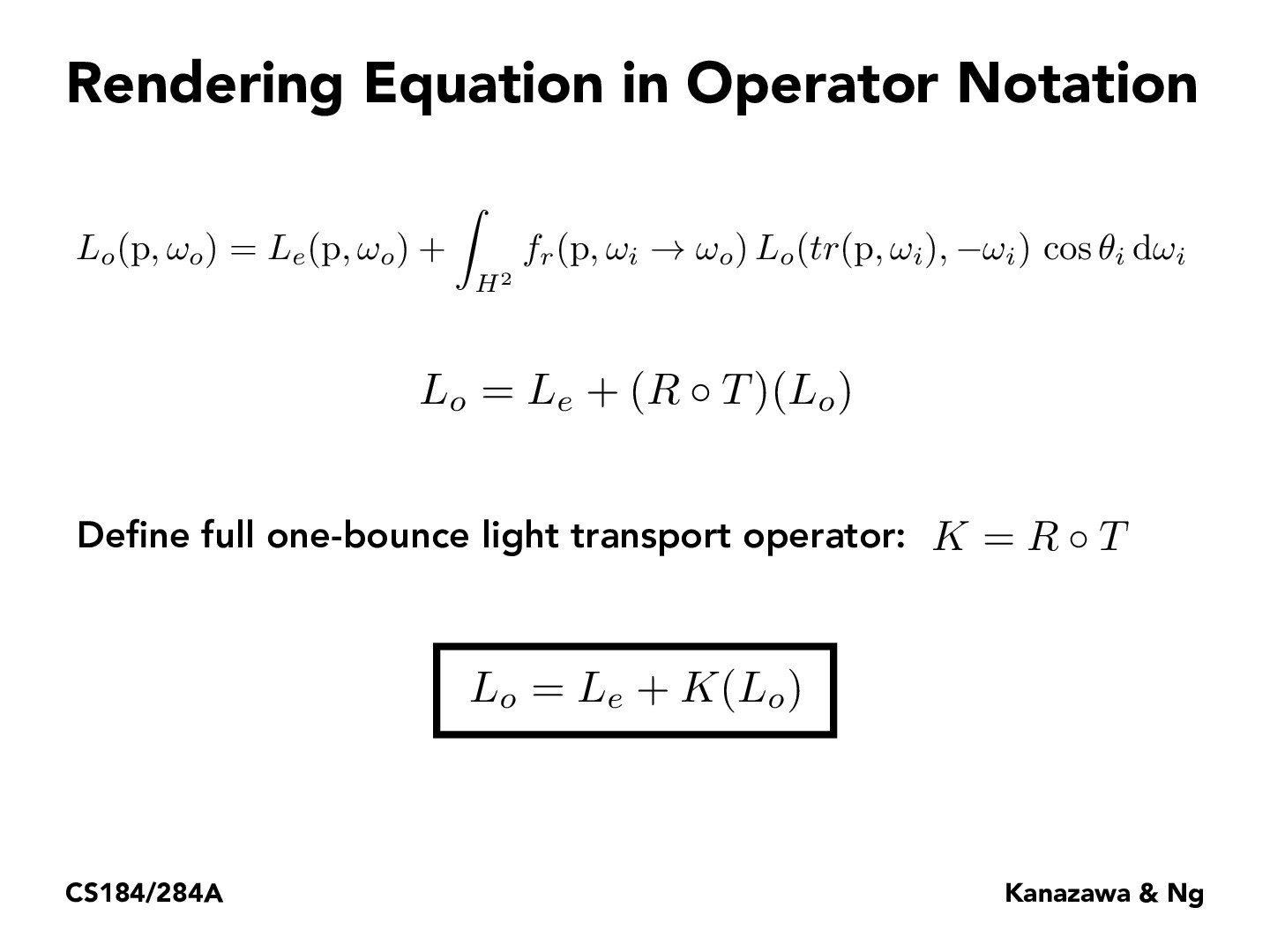Lecture 13: Global Illumination & Path Tracing (38)selinafeng

The equation $L_o = L_e + (R \circ T)(L_o)$ means taking the outgoing light in all directions ($L_o$), transporting it to the next surface that the light will arrive at ($T$), and then reflecting off of the surface depending its material properties ($R$). For one bounce, we only compose ($R \circ T$) once but we can compose the operators repeatedly to represent many bounces.

You must be enrolled in the course to comment